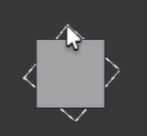# Move & rotate frame to cover area between points connected with line segments

Hey, so I was doing some physics stuff with guis and verlet integration. I was making rigid bodies for my physics engine. The way I have been going around till now is connecting points with line segments that act as constraints:

The red dots are the points and the black line segments are the constraints. Now the part where I am struggling is filling the empty area between these points and constraints.

I have found a solution for the same, which is using Gui Frames to determine shapes and sizes for the Rigid Bodies of the engine. Making 4 points on the corners of the Frame and not rendering any constraints or points. But the problem arises when correctly positioning this frame between the 4 points.

In the above image, if the 4 points change positions (while still being placed at certain positions which form rectangles), if I know the positions of the 4 points and the center point of the new rectangle, how would I go about positioning the Frame and rotating it correctly to fit perfectly to fill the space between the 4 points?

I can easily position the frame to the center point, but how would I go about rotating the Frame to cover the area perfectly?

I am guessing we’ll use trigonometry but don’t exactly know how to solve this problem. Any ideas?

lol dude this is just some simple rotational matrix. first get all your points into an array, then for each translate them to be origin then rotate them with a simple rotational transformation

``````local points = {}
local center = points["center"]
for i=1, #points do
local past = points[i].Position
points[i].Position = -center
local x = (points[i].Position.X*math.cos(k))-(points[i].Position.Y*math.sin(k))
local y = (points[i].Position.X*math.sin(k))+(points[i].Position.Y*math.cos(k))
points[i].Position = points[i].Position + Vector2.new(x,y)
points[i].Position = center
end
``````
1 Like

I think you misinterpreted my question, and sorry if my explanation wasn’t clear enough.

I have got the points to be rotating and moving correctly, I have no problem with that.

My problem lies in filling the area covered by this hollow body with a frame. If you know the area covered by this body as a Vector2, 4 corners of the body and its center, I can go ahead and create a frame. Set its anchor point to .5, .5 and position it to the body’s center. How would I rotate this frame to fit perfectly between the 4 points no matter where they are placed (whilst being constrained).

This is what I have till now:

In the picture below, the first shape is the Frame at rest. When I move it, the points move towards the right while maintaining uniform distance between each other (forming a hollow rectangle.). My task was to move the Old Rectangle frame and to place it correctly according to the points. (Indicated by the dotted line)

How it is (Ignore the cursor):How it should be:1 Like

well you can rotate guis with the rotation thing so just find the angle between the cube and when it has no rotation. form a vector from the center to one of the points then find the angle when it is rotated, change the fillers rotation to the opposite of that

1 Like

Hmm, I don’t quite get what you mean, wish to elaborate?

``````local rads = math.atan2((vertices.topLeft.pos - vertices.topRight.pos).y, (vertices.topLeft.pos - vertices.topRight.pos).x)